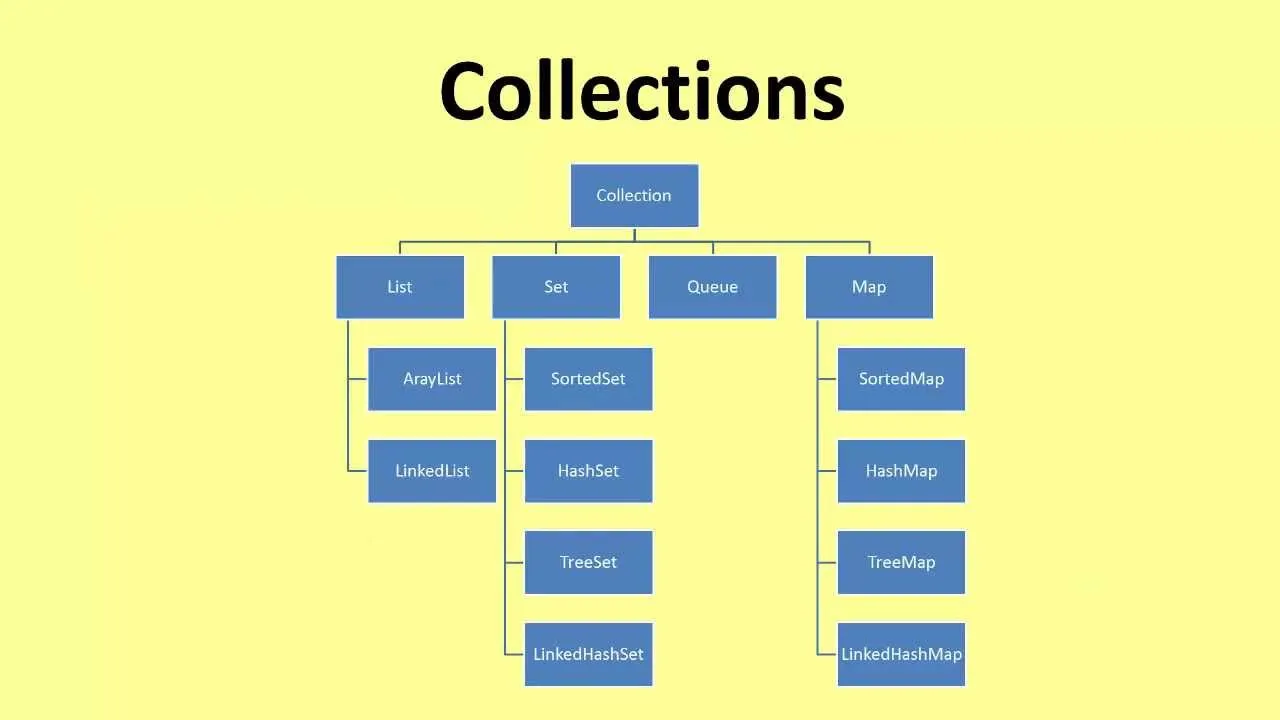# Programming - Java Data StructuresToday's post will be about implementing Data Structures in Java. The Data Structures in Java are already made Classes that we can use directly using an Object of this type or Interfaces that need implementation of specific functions we already seen in C. For Trees you have to write all the Code the same way we did in C. Here a Link that explains it pretty well: Binary Tree in Java

# Implementation:

To keep the Coding simple and small I will only use Classes and no Interfaces. All of those Classes are inside of the java.util library and the Object will contain all of the functions we already know how to use and even if printing doesn't make so much sense in things like Stacks or Queues I will show you a way I found works, even if we empty the Data Structure when doing so. So, without further do let's start things out.

## Node:

All of thoses Data Structures will contain a Node, a custom Datatype that will contain variables (int val, string s), constructors(default, overloaded) and 2 methods: toString() for printing and compareTo() for our PriorityQueue.

The Code looks like this:

``public class Node implements Comparable{ // Node.java``
`` // DATA``
`` int val;``
`` String s;``
`` // CONSTRUCTORS``
`` public Node() {``
``     val = 0;``
``     s = null;``
`` }``
`` public Node(int val, String s) {``
``     this.val = val;``
``     this.s = s;``
`` }``
`` // METHODS``
`` @Override``
`` public String toString() {``
``     return val + " " + s;``
`` }``
`` // function to check natural order``
`` @Override``
`` public int compareTo(Object o) {``
``     // cast to Node``
``     Node other = (Node)o;       ``
``     // call the compareTo String method``
``     return this.toString().compareTo(other.toString());     ``
`` }``
``}``

## List:

For List's you can use the ArrayList we already talked about as an List or you can use the LinkedList Object in the same exact way. The ArrayList is mostly faster and that's why you should use it. But, we already talked about it so I will show you how to create a LinkedList that uses those Nodes. Here some more stuff about it.

## Queue:

For creating a Queue I will use a PriorityQueue, cause it's already implemented with functions and so on. We need this compareTo() function we set up in our Node, cause a PriorityQueue uses some kind of Sorting. We will just compare the String representation of the Nodes (toString() method output) and put them in natural character order. For printing we can't just take an Item from an index, we will have to delete it, but setting it up using the Queue Interface you can make a custom Queue that works better. And, lastly the PriorityQueue should have some kind of value I guess for sorting or some kind of comparator function that makes more sense and checks some kind of priority key or number, if you want to use one in real. Here the Link to the PriorityQueue API doc.

## Stack:

An Stack is an already made Class that contains everything we need. We will use the push(), pop() and get(i) functions to do inserting, removing and printing with them. You can check the other ones out here.

# Code:

Lastly here a TestNodes class or Driver that will do all this stuff I talked about earlier in no time.

``import java.util.LinkedList;``
``import java.util.Stack;``
``import java.util.PriorityQueue;``
``public class TestNodes { // TestNodes.java``
`` public static void main(String[] args) {``
``                // Node Object for removing``
``     Node search;``

``     // LIST``
``     System.out.println("LIST:");``
``     // creating a List``
``                LinkedList<Node> list = new LinkedList<>();``
``     // add Nodes to list``
``     list.add(new Node(5, "hi"));``
``     list.add(new Node(3, "hey"));``
``     list.add(new Node(2, "holas"));``
``     list.add(new Node(7, "hello"));``
``     // remove Node from list``
``     search = list.removeFirst();``
``     System.out.println(search);``
``     search = list.removeLast();``
``     System.out.println(search);``
``     // print list``
``     for (int i = 0; i < list.size(); i++) {``
``         System.out.println(list.get(i));``
``     }``

``     // QUEUE``
``     System.out.println("QUEUE:");``
``     // creating a Queue of capacity 5``
````&nbspre;               PriorityQueue<Node> queue = new PriorityQueue<>(5);      `<re/p>
`     // add Nodes to queue in natural order`
`     queue.add(new Node(8, "bye"));`
`     queue.add(new Node(4, "bye bye"));`
`     queue.add(new Node(6, "adios"));`
`     queue.add(new Node(1, "goodbye"));`
`     // remove Nodes from queue`
`     search = queue.remove();`
`     System.out.println(search);`
`     search = queue.remove();`
`     System.out.println(search);`
`     // it doesn't contain a method for printing`
`     // and we can't get the i index so we remove`
`     // until queue is empty`
`     while(queue.peek() != null){`
`         search = queue.remove();`
`         System.out.println(search);`
`     }`

`     // STACK`
`     System.out.println("STACK:");`
`     // creating a Stack`
`          &nbw Stack);`
Stack&lt<>();
`     // add Nodes to stack`
`     stack.push(new Node(11, "what"));`
`     stack.push(new Node(9, "why"));`
`     stack.push(new Node(15, "who"));`
`     stack.push(new Node(12, "which"));`
`     // remove Nodes from stack`
`     search = stack.pop();`
`     System.out.println(search);`
`     search = stack.pop();`
`     System.out.println(search);     `
`     // print stack`
`     for (int i = 0; i < stack.size(); i++) {`
`         System.out.println(stack.get(i));`
`     }`
` }`
`}`

This was the end of today's Post. Hope you enjoyed it :)
Next time we will start out with GUI's in Java and we will talk about more Datastructures in C some day. I will upload more advanced Data Structures like Double LinkedLists, Priority Queues and AVL, Red-Black, 2-3 Trees in C.
```

H2
H3
H4
3 columns
2 columns
1 column